## Monday, December 2, 2019

### Representing (& graphing) the root edge of a tree as an additional unbranching edge

Yesterday a phytools user complained on Twitter that `phytools::plotTree` could not plot a root edge:

This would be easily functionality to add, but easier still would be to think about the root edge not as something special, but merely as an additional unbranching edge in the phylogeny.

The following function converts the root edge (normally stored as a separate part of the `"phylo"` object) to an additional edge in the edge matrix.

``````root.to.singleton<-function(tree){
if(!inherits(tree,"phylo"))
stop("tree should be object of class \"phylo\".")
if(!is.null(tree\$root.edge)){
tree\$edge[tree\$edge>Ntip(tree)]<-
tree\$edge[tree\$edge>Ntip(tree)]+1
if(attr(tree,"order")%in%c("postorder","pruningwise")){
tree\$edge<-rbind(tree\$edge,c(1,2)+Ntip(tree))
tree\$edge.length<-c(tree\$edge.length,tree\$root.edge)
} else {
tree\$edge<-rbind(c(1,2)+Ntip(tree),tree\$edge)
tree\$edge.length<-c(tree\$root.edge,tree\$edge.length)
}
tree\$root.edge<-NULL
tree\$Nnode<-tree\$Nnode+1
if(!is.null(tree\$node.label))
tree\$node.label<-c("",tree\$node.label)
}
tree
}
``````

Let's try it out:

``````library(phytools)
tree<-pbtree(n=64)
tree\$root.edge<-rexp(1)
plotTree(tree<-root.to.singleton(tree),ftype="off",
direction="upwards")
``````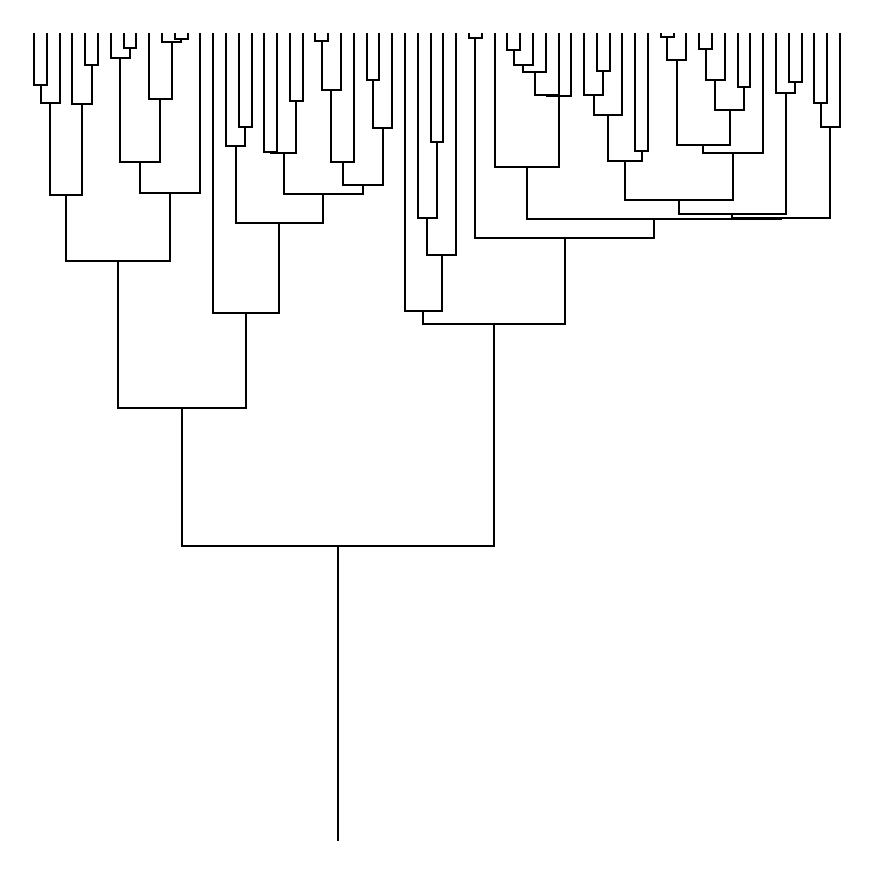The neat thing is that we can actually use this tree for other types of analyses too without any difficult - such as stochastic mapping. Let's try it.

``````## simulate some data
Q<-0.2*matrix(c(-1,1,1,-1),2,2,dimnames=list(c("a","b"),
c("a","b")))
x<-sim.Mk(tree,Q,anc="b")
x
``````
``````## t35 t36 t24 t21 t45 t46 t49 t54 t55 t27 t56 t59 t60  t5  t1 t13 t18 t19  t9 t10
##   b   b   b   b   b   b   a   a   a   a   a   a   a   a   b   b   b   b   b   a
## t25 t26 t57 t58 t32  t7 t39 t40 t16  t2  t4 t14 t15  t3 t61 t62  t6 t50 t51 t47
##   b   b   b   b   b   a   a   a   a   a   a   a   a   a   a   a   b   a   b   b
## t42 t29 t28 t30 t43 t44 t20 t11 t12 t63 t64 t48 t52 t53 t41 t33 t34  t8 t31 t37
##   b   b   b   b   b   b   b   b   a   b   b   b   b   b   b   b   b   a   b   b
## t38 t22 t23 t17
##   b   a   b   a
## Levels: a b
``````
``````## stochastic map
cols<-setNames(c("blue","red"),c("a","b"))
plot(make.simmap(tree,x),cols,ftype="off",lwd=3)
``````
``````## make.simmap is sampling character histories conditioned on the transition matrix
##
## Q =
##            a          b
## a -0.3206907  0.3206907
## b  0.3206907 -0.3206907
## (estimated using likelihood);
## and (mean) root node prior probabilities
## pi =
##   a   b
## 0.5 0.5
``````
``````## Done.
``````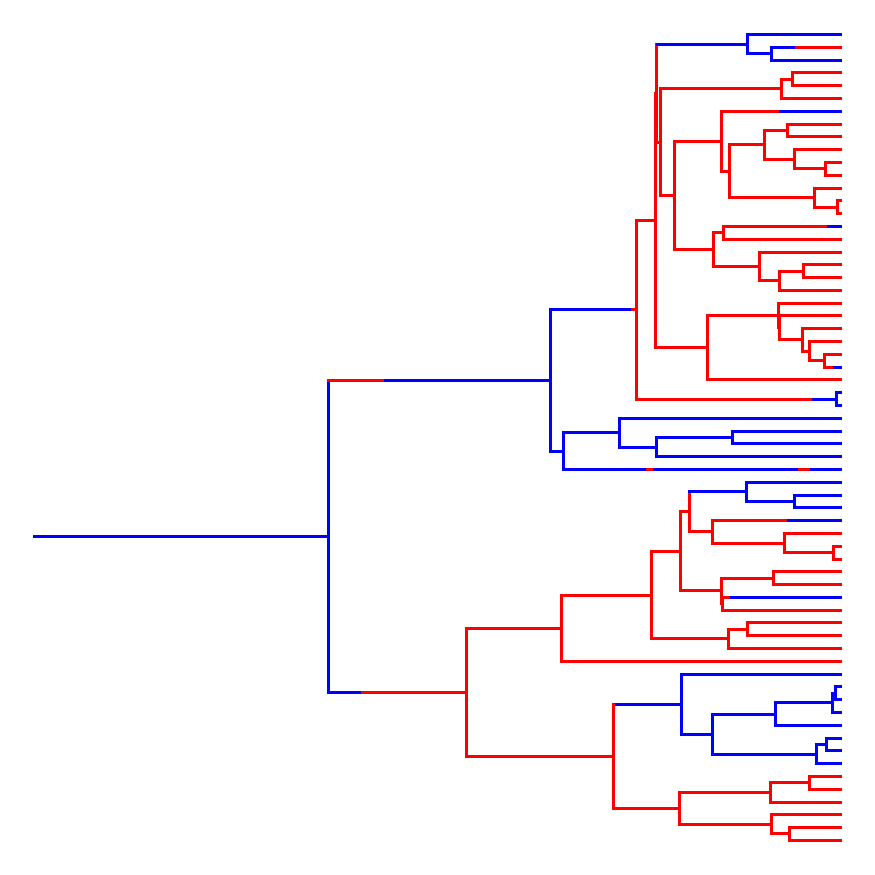``````## 100 stochastic character maps
tt<-make.simmap(tree,x,nsim=100)
``````
``````## make.simmap is sampling character histories conditioned on the transition matrix
##
## Q =
##            a          b
## a -0.3206907  0.3206907
## b  0.3206907 -0.3206907
## (estimated using likelihood);
## and (mean) root node prior probabilities
## pi =
##   a   b
## 0.5 0.5
``````
``````## Done.
``````
``````plot(summary(tt),colors=cols,ftype="off")
``````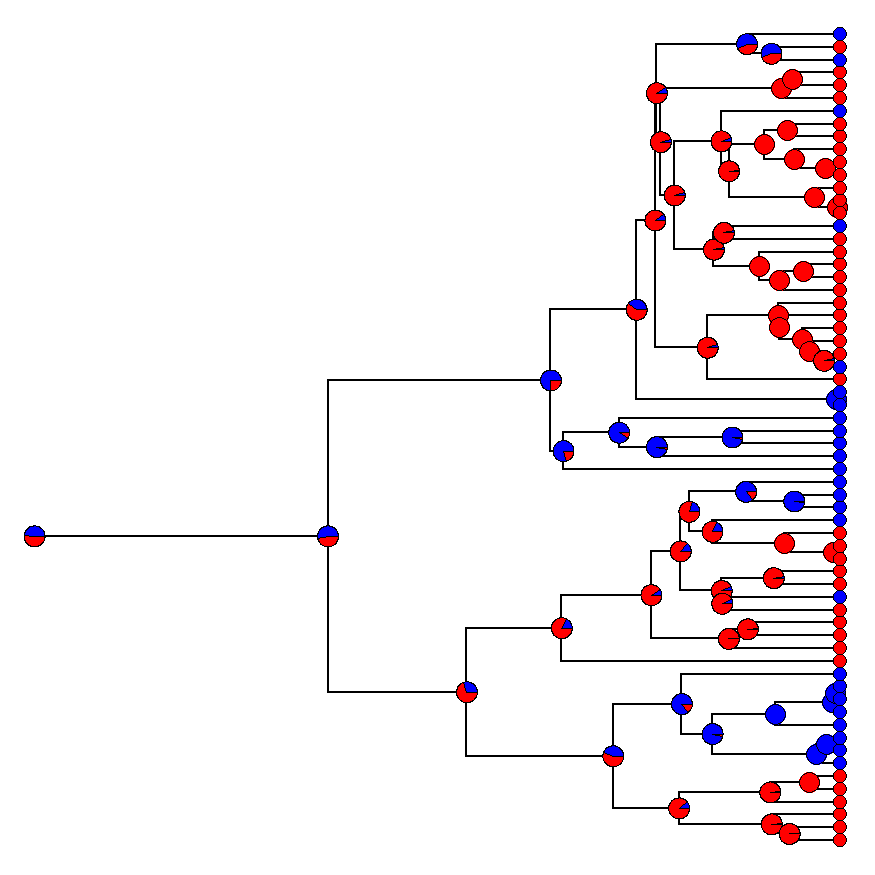``````## density map
densityMap(tt,ftype="off",lwd=c(4,8),res=200)
``````
``````## sorry - this might take a while; please be patient
``````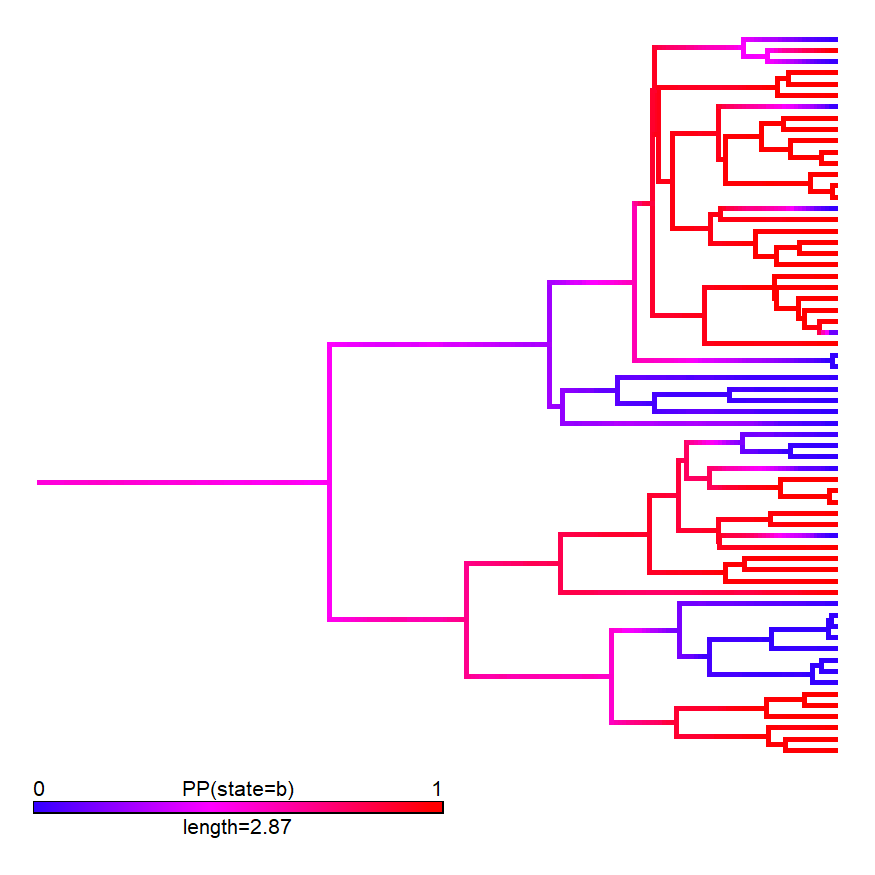Finally, this is also supported by other plotting styles as well, such as `type="fan"` trees:

``````plotTree(tree,type="fan")
``````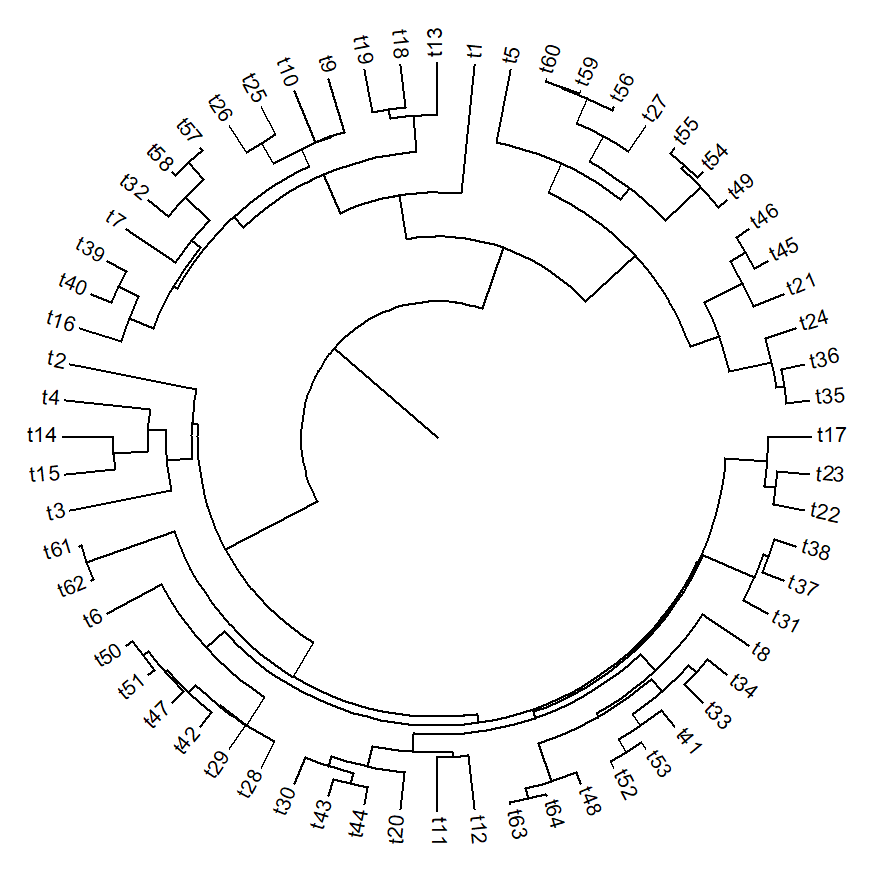I'll add `root.to.singleton` to phytools shortly.

1.1.